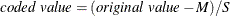Coding the Factor Variables

For the results of the canonical and ridge analyses to be interpretable, the values of different factor variables should be comparable. This is because the canonical and ridge analyses of the response surface are not invariant with respect to differences in scale and location of the factor variables. The analysis of variance is not affected by these changes. Although the actual predicted surface does not change, its parameterization does. The usual solution to this problem is to code each factor variable so that its minimum in the experiment is –1 and its maximum is 1 and to carry through the analysis with the coded values instead of the original ones. This practice has the added benefit of making 1 a reasonable boundary radius for the ridge analysis since 1 represents approximately the edge of the experimental region. By default, PROC RSREG computes the linear transformation to perform this coding as the data are initially read in, and the canonical and ridge analyses are performed on the model fit to the coded data. The actual form of the coding operation for each value of a variable iswhere M is the average of the highest and lowest values for the variable in the design and S is half their difference.